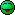# How tall is the bridge?

• keithcuda
In summary, the problem involves a rock being thrown horizontally from a bridge and hitting the water 30 meters away in 2 seconds. The formula used to solve for the height of the bridge is d=.5gt2, with g representing the acceleration due to gravity. The correct solution is 19.62 meters.

## Homework Statement

A rock thrown horizontally from a bridge hits the water below 30 meters away in the horizontal direction. If the rock was in the air for 2 seconds, how tall is the bridge?

d=.5gt2

## The Attempt at a Solution

d=.5*9.81*4
d= 19.62m
The bridge would be 19.62m high?

cheers,
Keith

It's been about 50 years since I've actually worked out a problem like that but it sounds wrong, and here's why: the acceleration due to gravity is 9.8 meters per second per second. That means that the rock will travel 9.8 meters in the first second, but much more in the next second, so the total can't be 9.8 x 2 which is what you got.

phinds said:
It's been about 50 years since I've actually worked out a problem like that but it sounds wrong, and here's why: the acceleration due to gravity is 9.8 meters per second per second. That means that the rock will travel 9.8 meters in the first second, but much more in the next second, so the total can't be 9.8 x 2 which is what you got.
It looks right to me. The first second it travels ~ 5 m, and the second second, it travels ~15 m.

Chet

•keithcuda
Chestermiller said:
It looks right to me. The first second it travels ~ 5 m, and the second second, it travels ~15 m.

Chet
Guess I have it wrong then that it travels 9.8m in the first second. I guess what the figure means is that at the end of the first second it is MOVING at 9.8 m/s, not that it has MOVED 9.8 m ... 50 years ago I bet I would have got it right